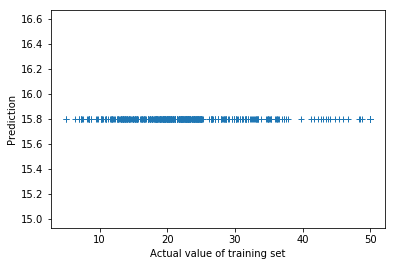# PyTorch fails to (over)fit Boston housing dataset

I am trying to use neural network to fit the boston housing dataset, as a starting point, I want to firstly overfit the training data. This seems to be a trivial task, the below code is used

``````from sklearn.datasets import load_boston
from sklearn.model_selection import train_test_split
from sklearn.neural_network import MLPRegressor
import numpy  as np
import sklearn
import matplotlib.pyplot as plt

import torch
import torch.nn as nn

X,y   = (boston.data, boston.target)
dim = X.shape

X_train, X_test, y_train, y_test = train_test_split(X, y, test_size=0.1, random_state=0)
num_train = X_train.shape

torch.set_default_dtype(torch.float64)
net = nn.Sequential(
nn.Linear(dim, 50, bias = True), nn.ReLU(),
nn.Linear(50,   50, bias = True), nn.ReLU(),
nn.Linear(50,   50, bias = True), nn.ReLU(),
nn.Linear(50,   1)
)
criterion = nn.MSELoss()
opt = torch.optim.Adam(net.parameters(), lr = 1e-6)

num_epochs = 1000
dataset = TensorDataset(torch.from_numpy(X_train).clone(), torch.from_numpy(y_train).clone())

for i in range(num_epochs):
loss = criterion(y, net(x))
loss.backward()
opt.step()
if i > 0 and i % 100 == 0:
print('Epoch %d, loss = %g' % (i, loss))

py = net(torch.DoubleTensor(X_train))
plt.plot(y_train, py.detach().numpy(), '+')
plt.xlabel('Actual value of training set')
plt.ylabel('Prediction')
``````

However, the trained model completely underfits the data, the below figure is drawn:This seems to indicate some bugs in my code instead of low model capacity, I also tried the `MLPRegressor` in sklearn using the very same architecture, optimizer and hyperparameters:

``````model = MLPRegressor(
hidden_layer_sizes=(50,50,50),
alpha = 0,
activation='relu',
batch_size=128,
learning_rate_init = 1e-3,
learning_rate = 'constant',
verbose = False,
n_iter_no_change = 1000,
validation_fraction = 0.0,
max_iter=1000)
model.fit(X_train, y_train)

py = model.predict(X_test)
err = y_test - py
mse = np.mean(err**2)
rmse = np.sqrt(mse)
print('rmse for test %g' % rmse)
plt.subplot(121)
plt.plot(y_test, py, '+')

err = y_train - model.predict(X_train)
mse = np.mean(err**2)
print('rmse for train: %g' % np.sqrt(mse))
plt.subplot(122)
plt.plot(y_train, model.predict(X_train), '+')
``````

The sklearn code gives reasonable predictions, as shown below:I found the reason, it’s because the shape of `y` and `net(x)` don’t match, so the MSE loss is incorrect

How to fix it??
Could you explain more?
I have similar problem.

In my problem, shape of `train_y` is `(455,)` , while the shape of `net(torch.from_numpy(X_train))` is `(455,1)` , the inconsistense of shapes would make the MSELoss incorrect.

For example, you can run the below code to see the difference:

``````import torch
import torch.nn as nn
xs   = torch.randn(100)
ys   = torch.randn(100)
crit = nn.MSELoss()
print('Loss1 = %g,  Loss2=%g' % (crit(xs, ys),  crit(xs.reshape(100, 1), ys)))
``````

Also you are missing the line that clears gradients, i.e., `opt.zero_grad()`.

1 Like

Thanks.

I have one more question!
Which shape is correct for MSEloss (455,) or (455,1)??

I think both shapes are OK, as long as they are the same.

Oh… It’s really interesting.
Why MSEloss distinct those two tensor?..

Anyway Thanks @Alaya-in-Matrix

It seems to be the broadcast semantics, if you define `MSE(x,y)` as `((x - y)**2).mean()`, you can see similar things in numpy

(455,) and (455,1) are different in broadcasting though, because broadcasting starts from the right end!

If you do (455,1) - (455,), you will get a (455, 455) tensor, while (455,1) - (455,1) gives you a (455,1) tensor. MSE, if it is implemented as (x - y).pow(2).mean(), will work differently.

1 Like

I’m facing similar issues with my model and noticed my targets are (400,) while outputs are (400,1). However, when changing the targets to (400,1) I still get the same MSELoss() that was calculated before. My predictions end up being a constant at the average of the targets. A similar implementation was done with SGD but with the same issue My code currently looks as such:

``````    for epoch in range(num_epochs):
MSE=0.0
input_data=data_sample
target=data_sample
batch_size=len(target)
target=target.reshape(400,1)

input_data=input_data.to(device)
target=target.to(device)

def closure():
output=model(input_data)
loss=criterion(output,target)
loss.backward()
return loss

loss=optimizer.step(closure)
MSE+=loss.item()*batch_size

MSE=MSE/dataset_size
RMSE=np.sqrt(MSE)
epoch_loss=RMSE
print epoch_loss
``````

This seems to work:
#!/usr/bin/env python

# coding: utf-8

from sklearn.model_selection import train_test_split
from sklearn.neural_network import MLPRegressor
import numpy as np
import sklearn
import matplotlib.pyplot as plt

import torch
import torch.nn as nn
import pandas as pd

X,y = (boston.data, boston.target)
dim = X.shape

X.shape

house.hist(column=‘medv’, bins=50)
plt.show()

# In:

X_train, X_test, y_train, y_test = train_test_split(X, y, test_size=0.9, random_state=0)
num_train = X_train.shape
X_train

# In:

torch.set_default_dtype(torch.float64)
net = nn.Sequential(
nn.Linear(dim, 50, bias = True), nn.ELU(),
nn.Linear(50, 50, bias = True), nn.ELU(),
nn.Linear(50, 50, bias = True), nn.Sigmoid(),
nn.Linear(50, 1)
)
criterion = nn.MSELoss()
opt = torch.optim.Adam(net.parameters(), lr = .0005)

# In:

num_epochs = 8000
y_train_t =torch.from_numpy(y_train).clone().reshape(-1, 1)
x_train_t =torch.from_numpy(X_train).clone()
#dataset = TensorDataset(torch.from_numpy(X_train).detach().clone(), torch.from_numpy(y_train).reshape(-1,1).detach().clone())
losssave = []
stepsave = []

for i in range(num_epochs):
y_hat = net(x_train_t)
loss = criterion(y_train_t,net(x_train_t))
losssave.append(loss.item())
stepsave.append(i)
loss.backward()
opt.step()
y_hat_class = (y_hat.detach().numpy())
accuracy = np.sum(y_train.reshape(-1,1)== y_hat_class )/len(y_train)
if i > 0 and i % 100 == 0:
print('Epoch %d, loss = %g acc = %g ’ % (i, loss, accuracy))

ss=np.array(stepsave)
ss.shape
sl =np.array(losssave)
sl.shape
#print (y_hat_class)
#print(y_train.reshape(-1,1))
#ss.reshape(8000)
#sl.reshape(8000)

# In:

py = net(torch.DoubleTensor(X_train))
plt.plot(sl, ‘+’)
plt.xlabel(‘Actual value of training set’)
plt.ylabel(‘Prediction’)
plt.show()

# In:

ypred = net(torch.from_numpy(X_test).detach())
err = ypred.detach().numpy() - y_test
mse = np.mean(err*err)
print(np.sqrt(mse))
plt.plot(ypred.detach().numpy(),y_test, ‘+’)
plt.show()

# In[ ]:

model = MLPRegressor(
hidden_layer_sizes=(50,50,50),
alpha = 0,
activation=‘relu’,
batch_size=128,
learning_rate_init = 1e-3,
learning_rate = ‘constant’,
verbose = False,
n_iter_no_change = 1000,
validation_fraction = 0.0,
max_iter=1000)
model.fit(X_train, y_train)

py = model.predict(X_test)
err = y_test - py
mse = np.mean(err2)
rmse = np.sqrt(mse)
print(‘rmse for test %g’ % rmse)
plt.subplot(121)
plt.plot(y_test, py, ‘+’)
plt.show()
err = y_train - model.predict(X_train)
mse = np.mean(err
2)

plt.plot(py)
py.mean()

# In[ ]:

Based on Anaya-In-Matrix## Wenlong Lyu’s jupyter notebook. The opt.zero_grad() was essential. Note batches were not used…this is a small problem.

Also the translation from/to numpy was a pain in the ass!

did you scale the data?